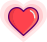• 给两个字符串，一长一短，问其中短的是否在长的中满足一定的条件存在，例如：

• 求长的的最短子串，该子串必须涵盖短的的所有字符

• 短的的 anagram 在长的中出现的所有位置

• 给一个字符串或者数组，问这个字符串的子串或者子数组是否满足一定的条件，例如：

• 含有少于 k 个不同字符的最长子串

• 所有字符都只出现一次的最长子串

//authour:P.yh//Editor:程序员小吴public int slidingWindowTemplate(String[] a, ...) {    // 输入参数有效性判断    if (...) {        ...    }    // 申请一个散列，用于记录窗口中具体元素的个数情况    // 这里用数组的形式呈现，也可以考虑其他数据结构    int[] hash = new int[...];    // 预处理(可省), 一般情况是改变 hash    ...    // l 表示左指针    // count 记录当前的条件，具体根据题目要求来定义    // result 用来存放结果    int l = 0, count = ..., result = ...;    for (int r = 0; r < A.length; ++r) {        // 更新新元素在散列中的数量        hash[A[r]]--;        // 根据窗口的变更结果来改变条件值        if (hash[A[r]] == ...) {            count++;        }        // 如果当前条件不满足，移动左指针直至条件满足为止        while (count > K || ...) {            ...            if (...) {                count--;            }            hash[A[l]]++;            l++;        }        // 更新结果        results = ...    }    return results;}

1

### 代码实现

// 使用上面的模板进行解题，so easy ！public List<Integer> findAnagrams(String s, String p) {    // 输入参数有效性判断    if (s.length() < p.length()) {        return new ArrayList<Integer>();    }    // 申请一个散列，用于记录窗口中具体元素的个数情况    // 这里用数组的形式呈现，也可以考虑其他数据结构    char[] sArr = s.toCharArray();    char[] pArr = p.toCharArray();    int[] hash = new int;    for (int i = 0; i < pArr.length; ++i) {        hash[pArr[i] - 'a']++;    }    // l 表示左指针    // count 记录当前的条件，具体根据题目要求来定义    // result 用来存放结果    List<Integer> results = new ArrayList<>();    int l = 0, count = 0, pLength = p.length();    for (int r = 0; r < sArr.length; ++r) {        // 更新新元素在散列中的数量        hash[sArr[r] - 'a']--;        // 根据窗口的变更结果来改变条件值        if (hash[sArr[r] - 'a'] >= 0) {            count++;        }        // 如果当前条件不满足，移动左指针直至条件满足为止        if (r > pLength - 1) {            hash[sArr[l] - 'a']++;            if (hash[sArr[l] - 'a'] > 0) {                count--;            }            l++;        }        // 更新结果        if (count == pLength) {            results.add(l);        }    }    return results;}

2

### 题目描述

输入: S = "ADOBECODEBANC", T = "ABC"输出: "BANC"

### 代码实现

// 使用上面的模板进行解题，受篇幅限制下面的代码就不添加注释了public String minWindow(String s, String t) {    if (s.length() < t.length()) {        return "";    }    char[] sArr = s.toCharArray();    char[] tArr = t.toCharArray();    int[] hash = new int;    for (int i = 0; i < tArr.length; ++i) {        hash[tArr[i]]++;    }    int l = 0, count = tArr.length, max = s.length() + 1;    String result = "";    for (int r = 0; r < sArr.length; ++r) {        hash[sArr[r]]--;        if (hash[sArr[r]] >= 0) {            count--;        }        while (l < r && hash[sArr[l]] < 0) {            hash[sArr[l]]++;            l++;        }        if (count == 0 && max > r - l + 1) {            max = r - l + 1;            result = s.substring(l, r + 1);        }    }    return result;}

3

### 题目描述

输入: "abcabcbb"输出: 3 解释: 因为无重复字符的最长子串是 "abc"，所以其长度为 3。

### 题目解析

• （1）如果当前遍历到的字符从未出现过，那么直接扩大右边界；

• （2）如果当前遍历到的字符出现过，则缩小窗口（左边索引向右移动），然后继续观察当前遍历到的字符；

• （3）重复（1）（2），直到左边索引无法再移动；

• （4）维护一个结果 res，每次用出现过的窗口大小来更新结果 res，最后返回 res 获取结果。

### 代码实现

public int lengthOfLongestSubstring(String s) {    if (s == null || s.length() == 0) {        return 0;    }    char[] sArr = s.toCharArray();    int[] hash = new int;    int l = 0, result = 1;    for (int r = 0; r < sArr.length; ++r) {        hash[sArr[r]]++;        while (hash[sArr[r]] != 1) {            hash[sArr[l]]--;            l++;        }        result = Math.max(result, r - l + 1);    }    return result;}

4

### 题目描述

输入: s1 = "ab" s2 = "eidbaooo"输出: True解释: s2 包含 s1 的排列之一 ("ba").

输入: s1= "ab" s2 = "eidboaoo"输出: False

### 代码实现

public boolean checkInclusion(String s1, String s2) {    if (s1.length() > s2.length()) {        return false;    }    char[] s1Arr = s1.toCharArray();    char[] s2Arr = s2.toCharArray();    int[] hash = new int;    for (int i = 0; i < s1Arr.length; ++i) {        hash[s1Arr[i] - 'a']++;    }    int l = 0, count = 0;    for (int r = 0; r < s2Arr.length; ++r) {        hash[s2Arr[r] - 'a']--;        if (hash[s2Arr[r] - 'a'] >= 0) {            count++;        }        if (r >= s1Arr.length) {            hash[s2Arr[l] - 'a']++;            if (hash[s2Arr[l] - 'a'] >= 1) {                count--;            }            l++;        }        if (count == s1Arr.length) {            return true;        }    }    return false;}

5
K 个不同整数的子数组

### 题目描述

（例如，[1,2,3,1,2] 中有 3 个不同的整数：1，2，以及 3。）

输出：A = [1,2,1,2,3], K = 2输入：7解释：恰好由 2 个不同整数组成的子数组：[1,2], [2,1], [1,2], [2,3], [1,2,1], [2,1,2], [1,2,1,2].

输入：A = [1,2,1,3,4], K = 3输出：3解释：恰好由 3 个不同整数组成的子数组：[1,2,1,3], [2,1,3], [1,3,4].

### 题目解析

[1,2,1,2,3]  // 窗口满足条件 l r         // 满足条件的子数组 [1,2][1,2,1,2,3]  // 窗口满足条件 l   r       // 满足条件的子数组 [1,2],[2,1],[1,2,1][1,2,1,2,3]  // 窗口满足条件 l     r     // 满足条件的子数组 [1,2],[2,1],[1,2,1],[1,2],[2,1,2],[1,2,1,2][1,2,1,2,3]  // 窗口不满足条件，移动左指针至满足条件 l       r   [1,2,1,2,3]  // 窗口满足条件       l r   // 满足条件的子数组 [1,2],[2,1],[1,2,1],[1,2],[2,1,2],[1,2,1,2],[2,3]

### 代码实现

public int subarraysWithKDistinct(int[] A, int K) {    if (A == null || A.length < K) {        return 0;    }    int[] hash = new int[A.length + 1];    int l = 0, results = 0, count = 0, result = 1;    for (int r = 0; r < A.length; ++r) {        hash[A[r]]++;        if (hash[A[r]] == 1) {            count++;        }        while (hash[A[l]] > 1 || count > K) {            if (count > K) {                result = 1;                count--;            } else {                result++;            }            hash[A[l]]--;            l++;        }        if (count == K) {            results += result;        }    }    return results;}

6

### 代码实现

public int characterReplacement(String s, int k) {    if (s == null || s.length() == 0) {        return 0;    }    char[] sArr = s.toCharArray();    int[] hash = new int;    int l = 0, maxCount = 0, result = 0;    for (int r = 0; r < sArr.length; ++r) {        hash[sArr[r] - 'A']++;        maxCount = Math.max(maxCount, hash[sArr[r] - 'A']);        while (r - l + 1 - maxCount > k) {            hash[sArr[l] - 'A']--;            l++;        }        result = Math.max(r - l + 1, result);    }    return result;}

END

##原 创 热 文 推 荐